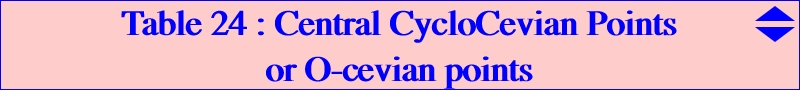Let M be a point and MaMbMc its cevian triangle. Any conic passing through Ma, Mb, Mc meets the sidelines of ABC again at Na, Nb, Nc. The triangles ABC and NaNbNc are perspective at N and the conic is said to be the bicevian conic C(M,N). More on bicevian conics in the Downloads page. We are here interested in the special case when M is the center of C(M,N). It is easy to see that, given M, there is a unique corresponding point N which is N = u / (v+w-u) : : , the barycentric quotient of M and its anticomplement. The conic is now called a central bicevian conic and is denoted C(M). Its equation is :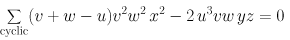C(G) is the Steiner in-ellipse and in this case N = M. Let us now denote by I(M) the inscribed conic with center M different of G. These two conics C(M) and I(M) are bitangent and the two contacts lie on the conjugated diameter in both conics of the trilinear polar of M. This line contains M and N.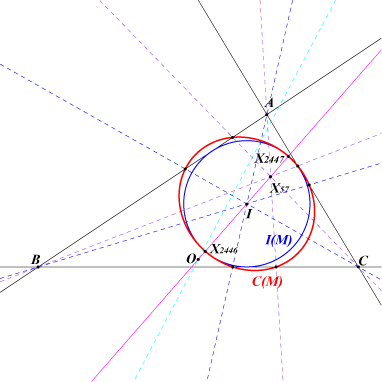Example : with M = I, I(M) is the incircle, C(M) is the conic passing through X(244), X(2170), X(2446), X(2447), X(2611), N = X(57), the contacts are X(2446), X(2447) on the line OI.C(M) and I(M) generate a pencil of bitangent conics which contains a circle if and only if M lies on the sextic Q055. We now seek the points M such that C(M) is a circle and naturally these points must lie on Q055. Moreover, M and N must be cyclocevian conjugates. If we express that C(M) is a circle, we find that M must be a common point of three quintics Q(A), Q(B), Q(C). The equation of Q(A) is given below and the other are obtained cyclically.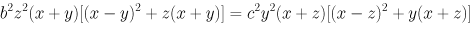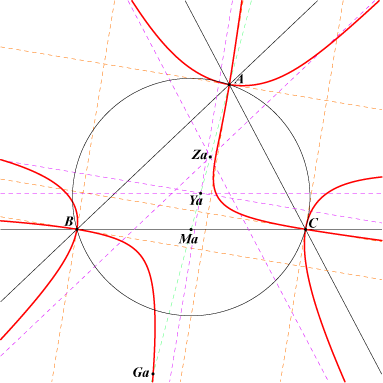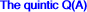Q(A) has three nodes A, B, C where the tangents are parallel to the bisectors at A. It contains Ga, the A-vertex of the antimedial triangle, and the tangent at this point passes through X(69), isotomic conjugate of H. It has five real asymptotes : three are parallel to the sidelines of ABC and two are parallel to the bisectors at A. Those parallel to AB, AC meet at Za, midpoint of the median AMa. The other concur at Ya, midpoint of Ma and Za.Since a^2 Q(A) + b^2 Q(B) + c^2 Q(C) = 0 for any x:y:z, we see that any common point of Q(B), Q(C) must lie on Q(A). It follows that the three quintics have 25 common points : A, B, C counting for 12, the infinite points of the sidelines counting for 6 (since the asymptotes parallel to the sidelines of ABC are common to the three quintics) and thus 7 remaining points Zx which we call central cyclocevian points or O-cevian points. In other words, a point is an O-cevian point if it is the circumcenter of its cevian triangle. One of these points is always real and the 6 other points might not be. This is generalized in : Table 28 : cevian and anticevian points. For any point P = p:q:r distinct of K, let us denote by Q(P) the quintic p Q(A) + q Q(B) + r Q(C) which obviously contains the seven points. With P complement of x:y:z, Q(P) decomposes into a circum-cubic (which cannot contain the 7 points since it has the same asymptotes as Q(A)) and the Lucas cubic K007. Hence we have the theorem : The Lucas cubic is the only circum-cubic containing the 7 central cyclocevian or O-cevian points.Recall that this cubic is invariant under isotomic and cyclocevian conjugations. It follows that the isotomic conjugate tZx and the cyclocevian conjugate oZx of any Zx must also lie on the Lucas cubic. Furthermore, the line through Zx and tZx passes through X(69), the pivot of the Lucas cubic. The figure shows the situation when only one Zx is real. Note that Q(A) and the Lucas cubic have 15 common points : the seven Zx and A, B, C, Ga (each one counting for two). One can obtain three real Zx with an almost "flat" triangle.Additional notes by Wilson Stothers Note 1 : If P is the complement of the isotomic conjugate of x:y:z, then Q(P) splits into (a) the Line at infinity, (b) the sidelines of ABC, and (c) the Lucas Cubic K007. Note 2 : If P = 0:1:1, then Q(P) splits into (a) the sideline BC, and (b) the quadric Q'(A). Q'(B) and Q'(C) are defined similarly. Note 3 : Q'(A)+Q'(B)+Q'(C) splits into (a) the Line at Infinity, and (b) the Lucas Cubic. Note 4 : The quadric Q'(A) (a) has a triple point at A, so Q'(A) is unicursal,     the real tangent at A is the median at A,     the other tangents at A pass through the infinite circular points, (b) contains B and C, so Q'(A) is a circumquadric, (c) has a real asymptote the parallel to BC at G,     this meets Q'(A) again on the angle bisectors at A, (d) contains the foot of the altitude at A. Note 5 : The O-cevian points lie on the Lucas Cubic and the quadrics Q'(A), Q'(B) and Q'(C). The intersection with Q'(A) has five intersections at A (triple), B and C, so there are indeed exactly seven O-cevian points. It needs to be checked that all also lie on Q'(B) and Q'(C). Note 6 : Since Q'(A) is unicursal, we can obtain the search numbers for these points. Note 7 : The isotomic conjugate of Q'(A) is a nodal cubic K(A). K(B) and K(C) are defined similarly. Then K(A)+K(B)+K(C) is the Lucas Cubic. The cubic K(A) (a) has a double point at A, so K(A) is unicursal,     the tangents at A are the side of the antimedial triangle at A, and the A-cevian of X69, (b) contains the foot of the A-median, (c) meets the circumconic with centre the mid-point of AG     on the A-cevian of X75, and     on the side of the anticevian triangle of X75 at A. Note 8 : The net of cubics identified in Note 7 gives yet another conjugation. The details appear very complicated - Maple cannot complete the necessary calculation. The only pair I know of is {X2,X253}. Note 9 : The net of quadrics generated by Q'(A), Q'(B), Q'(C) might be worth studying. For example, the members which pass through G also pass through X20, so we know all the intersections with the Lucas Cubic. After Note 3, we see that they must also have in common four points at infinity.